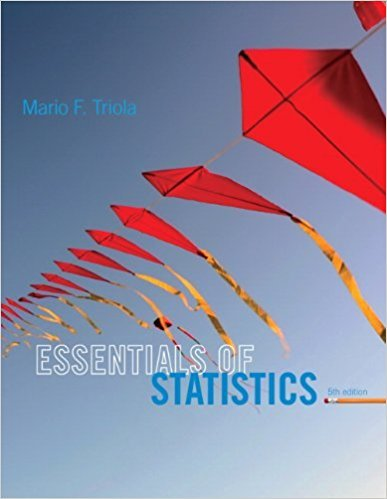×
×

# Scientific Notation. In Exercises 912, the givenISBN: 9780321924599 237

## Solution for problem 11 Chapter 1

Essentials of Statistics | 5th Edition

• Textbook Solutions
• 2901 Step-by-step solutions solved by professors and subject experts
• Get 24/7 help from StudySoup virtual teaching assistantsEssentials of Statistics | 5th Edition

4 5 1 277 Reviews
14
4
Problem 11

Scientific Notation. In Exercises 912, the given expressions are designed to yield re-sults expressed in a form of scientific notation. For example, the calculator-displayed re-sult of 1.23E5 can be expressed as 123,000, and the result of 4.56E-4 can be expressed as0.000456. Perform the indicated operation and express the result as an ordinary numberthat is not in scientific notation. 714

Step-by-Step Solution:
Step 1 of 3

Week 3 EDA for 2-Variable Data: - 2-variable data – when you have two measurements for each individual - An association (relationship) exists when values of a certain variable are more likely to occur when you have certain values of another variable - Explanatory...

Step 2 of 3

Step 3 of 3

##### ISBN: 9780321924599

This textbook survival guide was created for the textbook: Essentials of Statistics, edition: 5. The answer to “Scientific Notation. In Exercises 912, the given expressions are designed to yield re-sults expressed in a form of scientific notation. For example, the calculator-displayed re-sult of 1.23E5 can be expressed as 123,000, and the result of 4.56E-4 can be expressed as0.000456. Perform the indicated operation and express the result as an ordinary numberthat is not in scientific notation. 714” is broken down into a number of easy to follow steps, and 59 words. The full step-by-step solution to problem: 11 from chapter: 1 was answered by , our top Statistics solution expert on 01/12/18, 03:16PM. This full solution covers the following key subjects: . This expansive textbook survival guide covers 50 chapters, and 1368 solutions. Since the solution to 11 from 1 chapter was answered, more than 226 students have viewed the full step-by-step answer. Essentials of Statistics was written by and is associated to the ISBN: 9780321924599.

Unlock Textbook Solution